﻿ Hydrogen hyperfine structure, Fermi contact interaction

# Hyperfine structure by quantum mechanics is wrong.

## Hyperfine = electron nuclear spin interaction ?

### [ Schrodinger's 1s electron really "penetrates" nucleus ? ]

(Fig.1) Interaction between electron spin and nuclear spin ?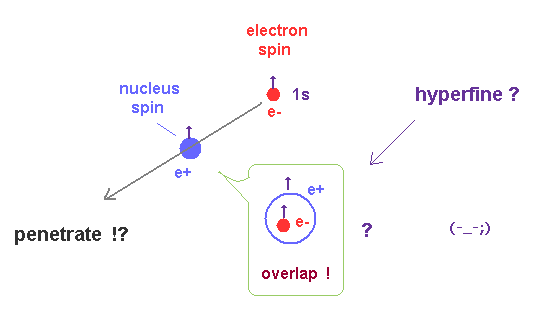This site says hyperfine structure is caused by the interaction between electron spin (= 1s ) and nuclear spin.
But the explanation by quantum mechanics is very strange and unrealistic.

They say Schrodinger's 1s electron is always penetrating proton (= nucleus ) !
Because the orbital angular momentum of "s" states is zero ( L = 0 ).

Suprisingly, only when this electron exists inside a small proton, hyperfine magnetic interaction (= 5.9 × 10-6 eV ) can occur, they insist.

Thinking commonsensically, it is impossible that such a small magnetic interaction can precisely happen inside proton.

## Quantum electrodynamics ( QED ) cannot predict nuclear parameters.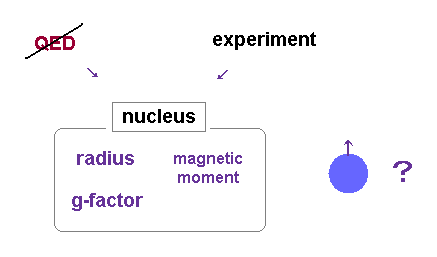As shown on this site, the recent experiment showed that quantum electrodynamics ( QED ) is inconsistent with the actual value.   ( Proton radius,   QED -- 0.877 fm,   vs.   Experiment -- 0.841 fm. )

Basically, we cannot directly know the electronic and magnetic distribution of nucleus (= proton ).
Experimental data are indispensable.   So, QED is NOT first principle at all.

QED just estimates proton's radius from the difference between the experimental Lamb shift ( ? ) and its prediction.   So, various webnews sites tend to "exaggerate" the meaning of "tricky" QED.

Furthermore, the nuclear magnetisation (= Bohr-Weisskopf correction ) is the main cause of uncertainty affecting QED, as shown on this site.

## Hyperfine by quantum mechanics (= QM ) is strange.

### [ Fermi contact interaction shows electron can "enter" nucleus !? ]

(Fig.3) Fermi contact accounts for the leading order of hyperfine structure.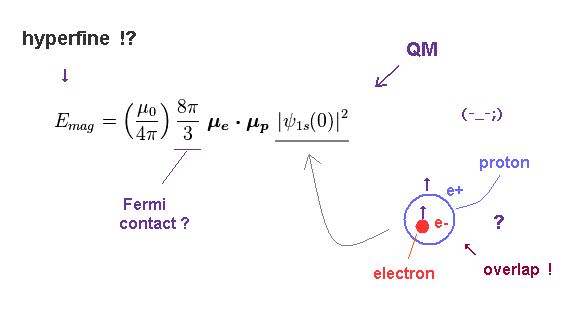It is said that Fermi contact interaction is the main cause of hyperfine structure.
The important point is that this Fermi contact value has NO physical meaning at all.

In Fig.3, μe and μp are magnetic moments of electron and proton.
The probability of ψ(0) means 1s electron can interact with nucleus only when it exists inside proton !

They just artificially adjust this primary Fermi contact by QED, as shown on this site (p.26).
So, the convenient QED is used only for small adjustment, though it gives infinity.

The problem is how this 8/3 π coefficient is gotten.
Unfortunately, there are NO convincing reasons for this value, as I say later.

## Nuclear magnetic field is produced Only inside proton !?

(Fig.4)   Quantum mechanics (QM) argues magnetic field exists only inside proton !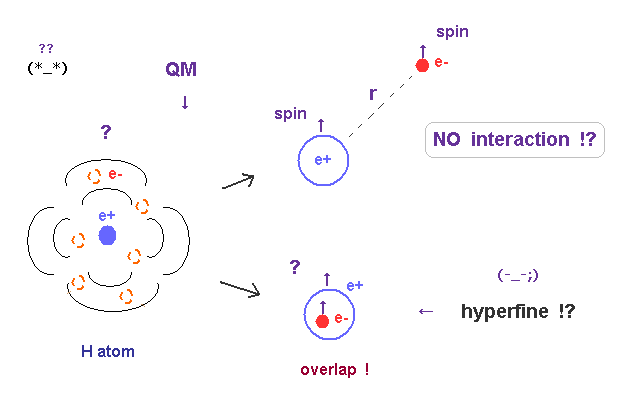According to Fig.3, they use only the electron probability at the origin (= nuclear position, r = 0 ).
This means the magnetic field is produced Only inside proton.

Of course, this is just a far-fetched interpretation with NO physical entity.
But they had to rely on the coefficient of this ψ(0) to get hyperfine value, though it lacks reality.

Thinking commonsensically, extremely weak hyperfine energy (= 5.9 × 10-6 eV ) is easily disturbed and gone in the circumstances filled with huge Coulomb force and other effects inside a proton.

## Electron spinning speed is much faster than light !

(Fig.5) Electron spin enters proton → spinning speed is faster than light.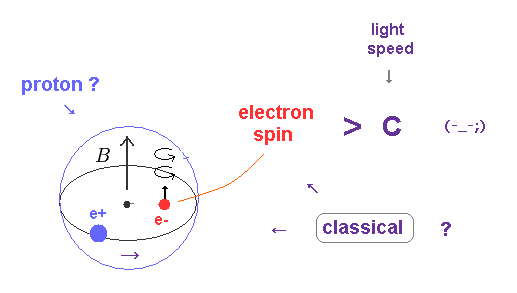The quantum mechanics argues the whole electron must enter the proton, and interact with proton's magnetic field (= B ).
As shown on this page, electron spinning speed becomes 1644 × 108 m/s (= more than 500 times light speed ! ) inside proton !

So the idea that electron inside proton causes hyperfine structure is unrealistic and impossible.

## Probability density at the nucleus is zero.

(Fig.6)   Electron's probability at the origin (= nucleus ) is zero.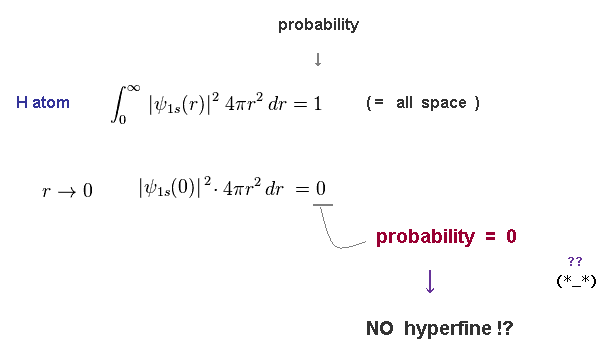Electron's probability is given by wavefunction × radius (= r ).
This means the electron's probability at the origin (= nucleus ) becomes zero !

So the hyperfine structure itself cannot occur according to Schrodinger's hydrogen ?
( Lamb shift by QED is impossible, too. )

## Magnetic field (= B ) at the proton diverges to ± infinity !?

(Fig.7) Magnetic field near the origin (= proton ) becomes infinite.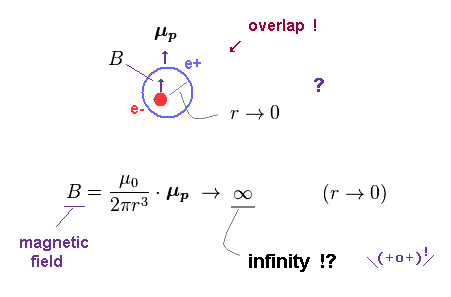According to this site (p.2) and this page, the magnetic field (= B ) generated by the magnetic moment (= μp ) is inversely proportional to the third power of r (= distance ).

When the electron comes near the proton, the distance between them is closer to zero ( r → 0 ).
As a result, their magnetic energy ( field ) diverges to ± infinity !

## Magnetic field of Fermi contact diverges to "infinity" at r = 0.

(Fig.8)   Unrealistic magnetic field in hyperfine structure.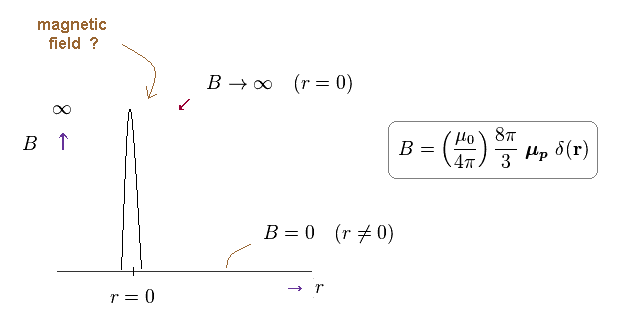As shown on this site (p.2), the magnetic field in the hyperfine structure is expressed using Dirac delta function (= δ(r) ).
So the magnetic field diverges to infinity at the origin (= nucleus ) !

They argue this infinite magnetic field B can cancel the zero probability of electron at the origin (= Fig.6 ).
Because (= B ) × 0 (= probability ) at the origin becomes some "finite" value.

As you see, this idea of quantum mechanical hyperfine structure is very ad-hoc and too good to be true.

## Energy distribution by quantum hyperfine is strange.

(Fig.9) Magnetic energy becomes ±∞ only at the origin !?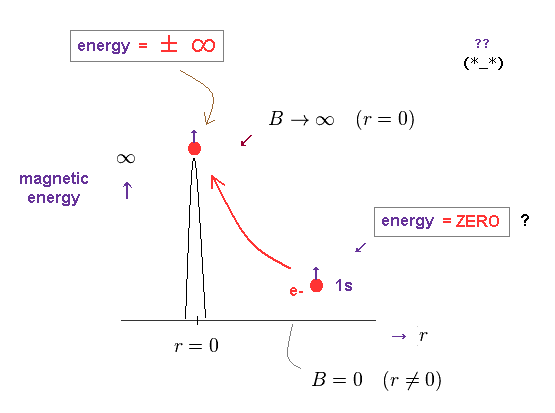Using the magnetic field (= B ) based on delta function, we can know magnetic energy distribution in the hyperfine state.
Surprisingly, this magnetic energy diverges to ±∞ at the origin.

In all other places, this magnetic energy always becomes zero !
How this electron can absorb or emit high energy photons depending on its position !?   ← Impossible.

## How big is this magnetic dipole energy inside proton ?

### [ Hyperfine structure by quantum mechanics needs unrealistically "big" energy. ]

(Fig.10) Magnetic dipole energy becomes 3 × 108 eV inside proton !   Impossible !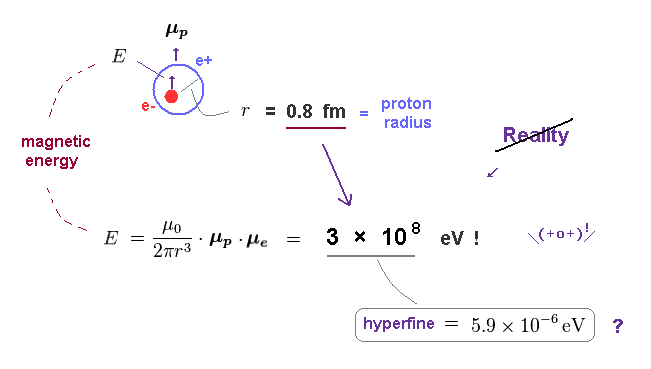Using the proton's radius (= 0.8 fm = 0.8 × 10-15 m ), the magnetic dipole energy ( see Fig.7 ) becomes as big as 3 × 108 eV inside proton !

It is impossible that such a big magnetic energy (= 3 × 108 eV ) suddenly appears and is given to ( or emitted from ) electron within a range of extremely small hyperfine state (= 5.9 × 10-6 eV )

## Schrodinger's wavefunction includes Bohr radius.

(Fig.11) 1s hydrogen wavefunction contains Bohr radius (= a0 ).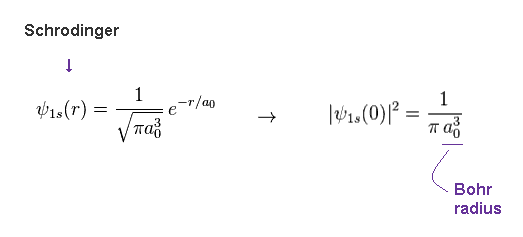As shown on this site (p.3), the hydrogen 1s wavefunction contains the reciprocal of the third power of Bohr radius (= 1/a03 ).

So, in fact, the probability at the origin possesses the information of the mean distance between electron and nucleus, like classical model of this page.

This is the important trick in hyperfine structure by quantum mechanics.

## Prediction of hyperfine structure by simple model.

(Fig.12) Magnetic interaction between nuclear spin and Bohr magneton ?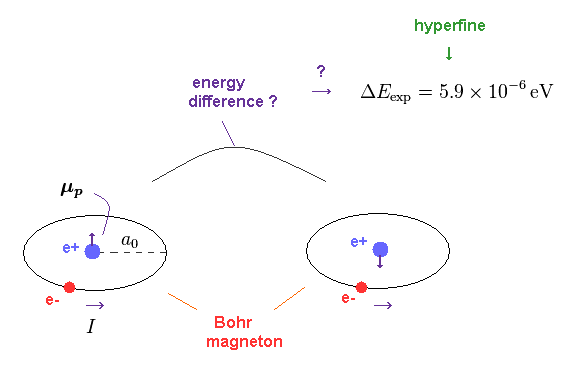First we try to calculate the magnetic interaction between nuclear spin (= μp ) and electron's magnetic moment (= Bohr magneton. )

Bohr magneton was first obtained using Bohr's classical orbit.
Accidentally, the magnetic dipole moment of electron spin is just equal to this Bohr magneton !

The magnitude of proton's magnetic moment is too small in comparison with electron spin.
( Proton magnetic moment = about 1/1000 × electron magnetic moment. See this site. )

Considering finite proton radius (= 0.8 fm ) and heavy mass, the spinning speed of proton does NOT exceed the light speed, as shown on this page.

## Magnetic field created by classical magnetic moment.

(Fig.13)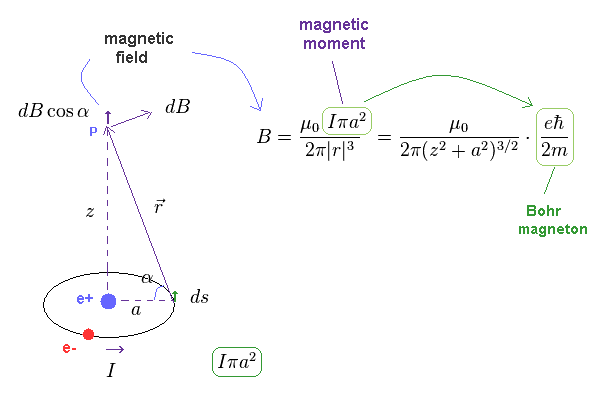As shown on this page, the magnetic field (= B ) generated by classical magnetic moment (= Iπa2 ) becomes like Fig.13.   Magnetic moment is given by the area × current I.

Here, electron (= e- ) is moving in the circular orbit, which generates electric current I.
"B" is the magnetic field at the point "p".

(Fig.14) Magnetic field caused by circular current I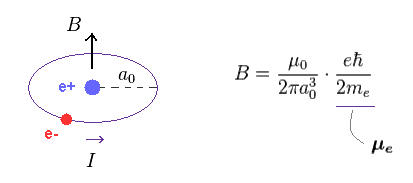When z = 0 in Fig.13, the magnetic field B at the nucleus (= e+ ) becomes Fig.14.
As I said, this magnitude is inversely proportional to the third power of the distance (= a0 ).

(Fig.15) Bohr magneton = electron magnetic moment.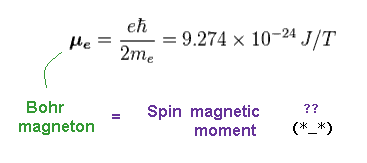e" is Bohr magneton, which is just equal to electron ( spin ) magnetic moment.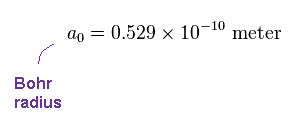"a0" is Bohr radius (= 0.529 Å ), which is the mean radius of hydrogen ground state.

(Fig.17) Proton spin magnetic moment (= μp ) is much smaller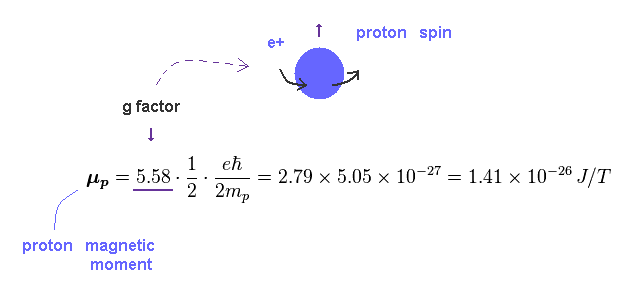Proton's magnetic moment (= μp ) is much smaller (= 1/700 ) than electron due to its heavy mass (= mp ).

The important point is that proton's g factor (= 5.58 ) is an experimental (= NOT QED ) parameter, which is different from Dirac equation.   Dirac equation gives only "2"

## What is hyperfine ? --- From "realistic" viewpoint.

(Fig.18) Interaction between electron-nuclear spins by simple model.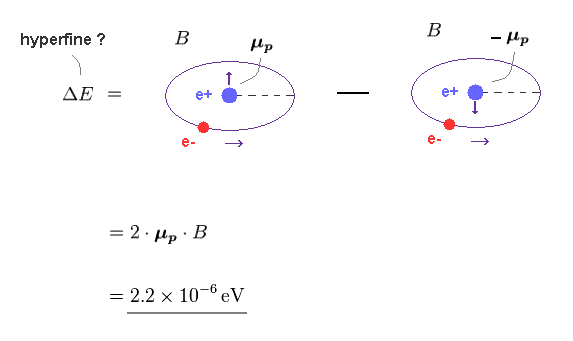Using Fig.14 - Fig.17, we can know the energy difference between "up" and "down" nuclear spins.
This energy interval becomes 2.2 × 10-6 eV

(Fig.19)   Hyperfine structure.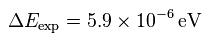Experimental value of hyperfine structure is 5.9 × 10-6 eV.
So, these values have almost same order ( ~ 10-6 eV ), but there is small difference.

### [ Hyperfine structure = the mix of proton spin, cyclotron and vibration. ]

(Fig.20) Very weak hyperfine structrue is susceptible to other small effects.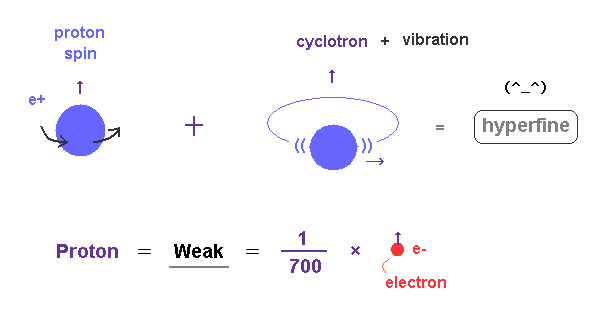Proton's magnetic moment is extremely small ( about 1/700 × electron magnetic moment ).
So the very weak hyperfine structure is easily affected by other small effects such as nuclear cyclotron and vibration.

First, proton itself is always rotating and moving by the electron's attractive force (= reduced mass ).
It is quite natural that the proton has small energy difference depending on its rotating directions due to "Magnus" effect or something.

## Hyperfine structure by quantum mechanics is unreal.

(Fig.21) Only when electron is inside proton, it can interact with proton ?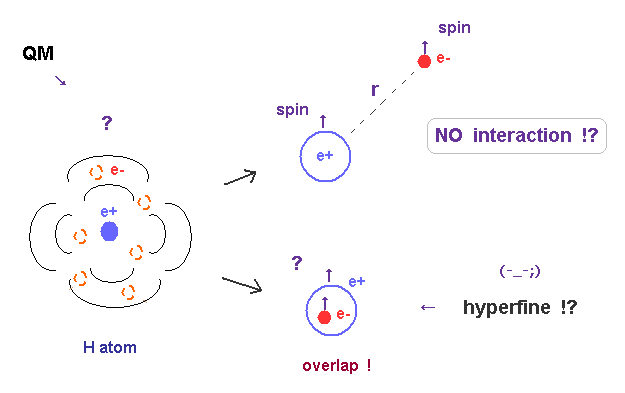On the other hand, the hyperfine model by quantum mechanics is crazy.
They argue that the electron can interact magnetically with proton, only when it exists inside proton !

(Fig.22)   Fermi contact is the leading term.According to quantum mechanics and this site, Fermi contact interaction (= part of delta function ) is the main factor of 1s hyeperfine, they insist.

## Fermi contact is based on classical electromagnetism !?

(Fig.23) Magnetic field (= B ) created by circular current I.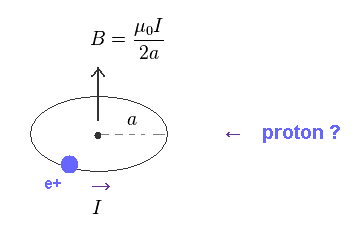The important point is that there are NO clear reasons for this Fermi contact value (= 8/3π ).
For example, relativistic Dirac equation can only give g-factor = 2 (← NOT proton ) in all fermions.

Surprisingly, "Classical Electrodynamics" ( J.D. Jackson ) says this Fermi contact is based purely on classical electromagnetism !   See this site. So quantum mechanical "spin" is illusion ?

As shown on this site, the magnetic field B produced by circular electric current I is given by Fig.23.

## "Classical" Fermi contact interaction ?

(Fig.24) The total magnetic field included inside proton's sphere ?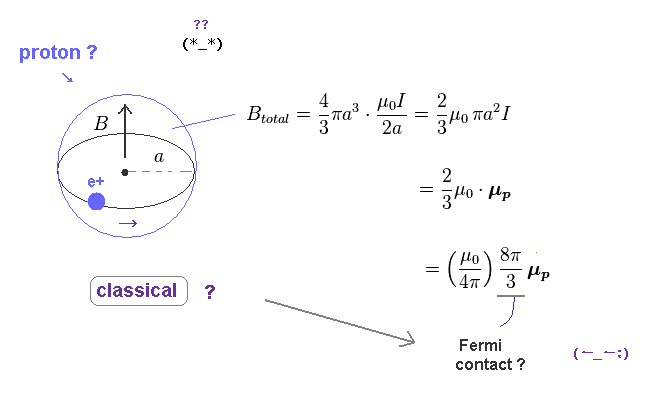Next, they integrate the magnetic field of Fig.23 over the volume of the sphere (= 4/3πa3 ) to get total magnetic field.
This value of "4/3" related to sphere is the key point.

As a result, this total magnetic field inside the proton's sphere gives Fermi contact term of Fig.22, they insist.
As you see, this calculation is based purely on classical ( NOT quantum ) mechanics.

(Fig.25) Other magnetic field is cancelled out.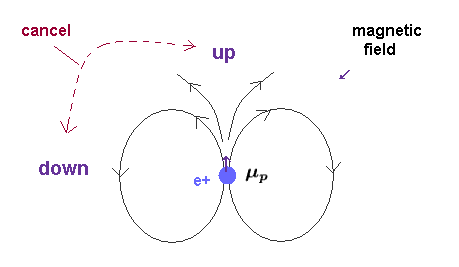Basically the magnetic field is "closed" system.
So the total magnitudes of "up" and "down" magnetic fields are the same in all space.

If you integrate the magnetic field outside the proton's sphere, you will find the total magnetic field becomes zero.
This means "spin-spin" magnetic interaction ( ex. triplet, singlet ) cannot happen according to this logic.

## All proton's magnetic field concentrate into the origin ? → delta function.

(Fig.26) Dirac delta function (= δ ).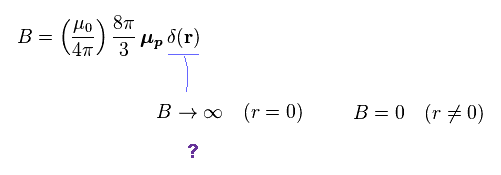More suprisingly, they concentrate that total magnetic field into the one single point ( r = 0 ) !
So, the magnetic density (= B ) at the origin becomes infinite !

There are No magnetic fields in other places, they insist.
Of course, this situation is completely out of touch with reality.

## Rigorous derivation of total magnetic field.

(Fig.27) Magnetic field B is given by the curl of vector potential A.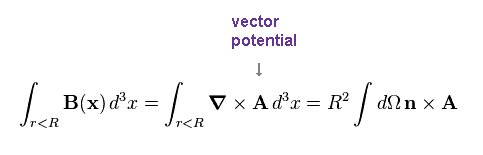As shown on this site, the magnetic field B is defined by the curl of vector potential A.
This vector potential is defined to be consistent with classical Ampere's ( Biot-Savart ) Law.

They integrate the magnetic field B over the volume of proton's sphere. (← Trick ! )
"R" is the radius of the proton's sphere.

(Fig.28)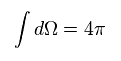"Ω" is solid angle (= 4π ) in the spherical coordinate.

## Classical Biot-Savart law is valid even "inside" a proton !?

(Fig.29) Biot-Savart law = "classical" electromagnetism !?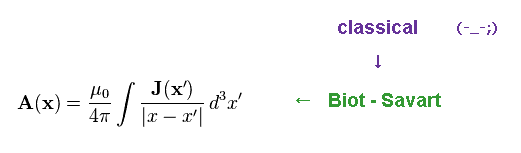They use the definition of vector potential "A" based on classical electromagnetism.
The relation of Fig.29 gives classical Bio-Savart law. See this site (p.11)

(Fig.30) Total magnetic field.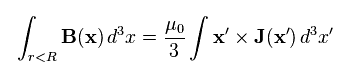After the long complicated calculation using Fig.27 - Fig.29, they get Fig.30.
The important point is that this method completely depends on the assumption that the proton is a pure sphere.

(Fig.31) Magnetic moment μp of proton.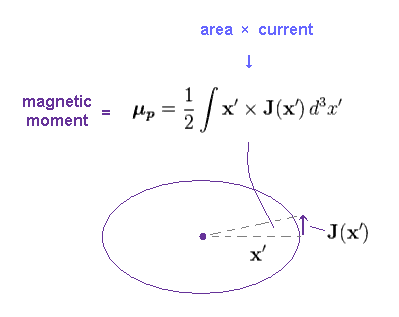The magnetic moment μ is given by the area × current.
The integral of Fig.31 expresses the total magnetic moment inside proton.

(Fig.32) Total magnetic field → Fermi contact ?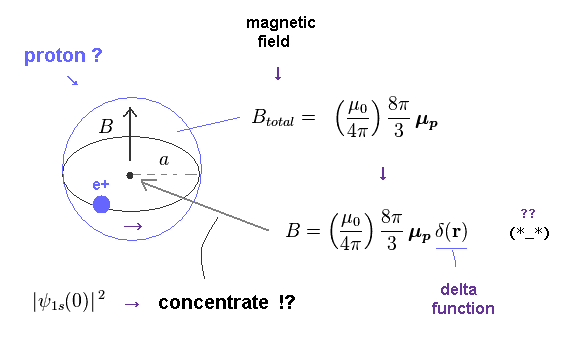From Fig.30 and Fig.31, we obtain the total magnetic field of Fig.32.
And they concentrate this total B only into the single point ( r = 0 ) using Dirac delta function.

This is also one of artificial tricks.
As a result, they can get Fermi contact term of Fig.22.

## "Classical" current → electron spin is much faster than light !?

(Fig.33) Tiny electron spinning speed is superluminal !?They derived the Fermi contact term using purely classical mechanics (= circular current + Biot-Savart ).
If classical mechanics dominates even inside proton, the electron spin magnetic moment must be classical, too.

But as shown on this page, the electron spinning speed is much faster than light ( > c ), when its rotating radius is equal to that of a proton.
( Of course, an electron is much smaller, so its speed becomes much faster ! )

(Fig.34) Magnetic field outside proton is cancelled out.As I said, the integral of the magnetic field over the space (= sphere ) outside the magnetic moment becomes zero.
Because the magnitudes of "up" and "down" magnetic field are just the same.

So the total magnetic field in all space becomes Fig.32.
Of course, this value changes depending on the electric current's shape.   ex. circular → elliptic.

This means this obtained value is "model-dependent".

## We can "arbitrarily" designate the magnetic field area.

(Fig.35) We can arbitrarily choose the magnetic field area.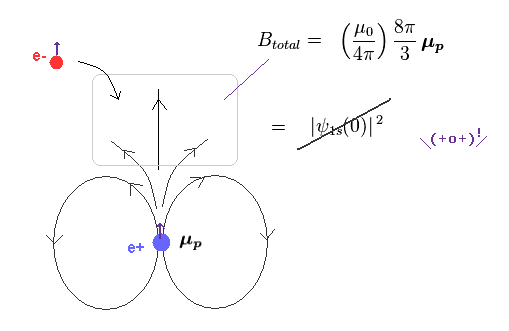In Fig.34, I said the total magnetic field outside proton is cancelled out.
But of course, the magnetic density in each area is NOT zero.

So if we choose other areas as contributing to the total magnetic field of Fig.32 instead of the origin, the probability density of electron's wavefunction (= ψ ) in those areas changes. (= NOT ψ(0) ! )

As a result, the resultant hyperfine structure changes depending on this chosen area.
This means that this method cannot give the definite value as a hyperfine structure, and we can arbitrarily change that value by designating magnetic areas.

(Fig.36) Calculated value is still different from experimental value.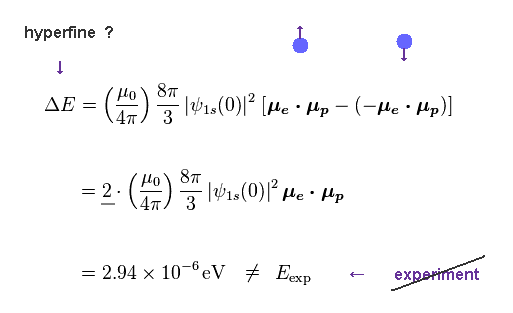The hyperfine structure expresses the energy difference between "up" and "down" nuclear spins.
As shown in Fig.36, this value based on classical Biot-Savart law cannot give correct hyperfine structure.

So, they had to use other artificial tricks after classical calculation.

## Artificial trick.

(Fig.37) Electron (= S ) and nuclear (= I ) spins.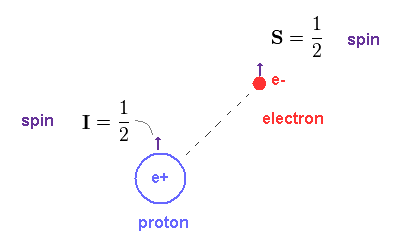Here "S" is electron spin angular momentum (= 1/2 ), and "I" is nuclear spin (= 1/2 ).
The ratio of magnetic moment to angular momentum (= 1/2 ) is called "quantum" g-factor. See this site.

(Fig.38)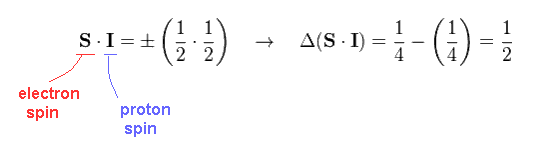So the product of S mutiplied by I is 1/2 × 1/2 = 1/4.
The different between ±1/4 becomes 1/2, in this case.

(Fig.39)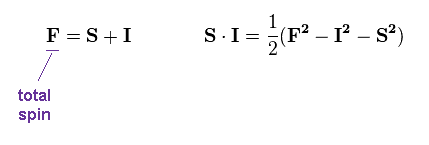Here they define total spin (= F ).

## Which is true, classical or quantum !?

(Fig.40) ↓ Quantum effect ?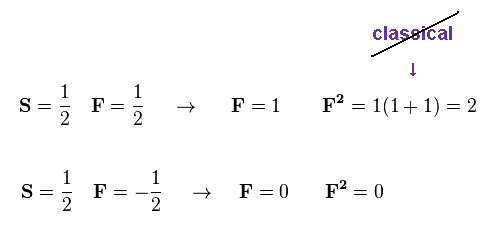According to the quantum mechanics, the square of F becomes "2", even when F = 1.

(Fig.41)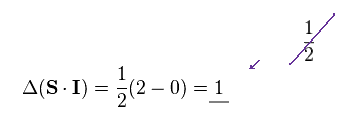As a result, the difference in ±"SI" becomes twice (= 1 ) the classical value.
( Compare "1" in Fig.41 with "1/2" in Fig.38. This is the last trick. )

## After "classical" magnetic field is calculated, "quantum" effect can be used ?

(Fig.42) Artificial trick.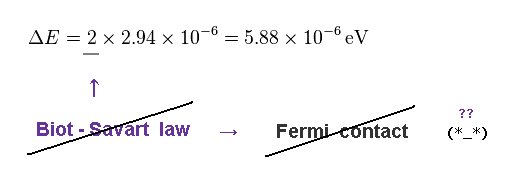As a result, they can get the value close to experimental hyperfine structrue.
But this method is very ad-hoc, and inconsistent.

As shown in Fig.29, they get the total magnetic field value, depending on purely classical Biot-Savart law.
In spite of this, they completely ignore this definition, and intentionally double the magnetic field using quantum's trick.

Thinking commonsensically, if the last manipulation based on quamtum mechanics is right, calculation using classical electromagnetism itself becomes invalid and cannot be used in the first step.2014/10/13 updated. Feel free to link to this site.